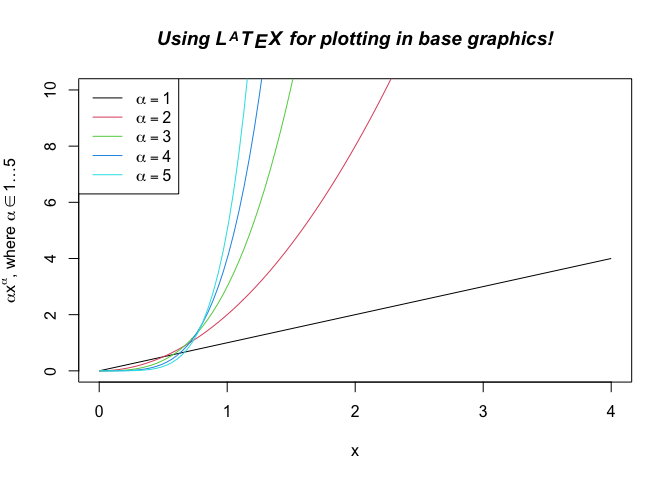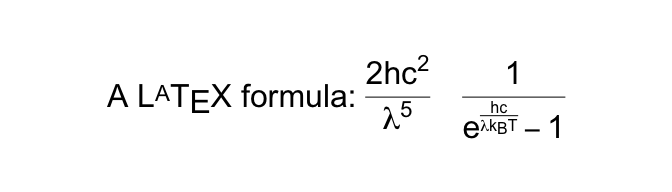# latex2exp

latex2exp is an R package that parses and converts LaTeX math formulas to R’s plotmath expressions. Plotmath expressions are used to enter mathematical formulas and symbols to be rendered as text, axis labels, etc. throughout R’s plotting system. I find plotmath expressions to be quite opaque, while LaTeX is a de-facto standard for mathematical expressions, so this package might be useful to others as well.

## Installation

Install this package from CRAN:

install.packages('latex2exp')

You can also install from GitHub using devtools:

devtools::install_github('stefano-meschiari/latex2exp')

## Usage

library(latex2exp)

The TeX function takes a LaTeX string and returns a plotmath expression suitable for use in plotting, e.g.,

TeX('$\\gamma^\\alpha$')

Before R 4.0, it is necessary to escape the backslash within the string literal: therefore, one writes '$\\gamma$' rather than '$\gamma$' (the latter will cause an error).

After R 4.0, it is recommended to use the new raw string literal syntax (see ?Quotes). The syntax looks like r"(...)", where ... can contain any character sequence, including \:

TeX(r'($\gamma^\alpha$)')

The return value of TeX() can be used anywhere a plotmath expression is accepted, including plot labels, legends, and text.

The following example shows plotting in base graphics:

x <- seq(0, 4, length.out=100)
alpha <- 1:5

plot(x, xlim=c(0, 4), ylim=c(0, 10),
xlab='x', ylab=TeX(r'($\alpha x^\alpha$, where $\alpha \in 1 \ldots 5$)'),
type='n', main=TeX(r'(Using $\LaTeX$ for plotting in base graphics!)', bold=TRUE, italic=TRUE))

invisible(sapply(alpha, function(a) lines(x, a*x^a, col=a)))

legend('topleft', legend=TeX(sprintf(r'($\alpha = %d$)', alpha)),
lwd=1, col=alpha)This example shows plotting in ggplot2:

library(purrr)
library(ggplot2)
library(tibble)

x <- seq(0, 4, length.out=100)
alpha <- 1:5
data <- map_df(alpha, ~ tibble(v=.*x^., x=x, alpha=.))

p <- ggplot(data, aes(x=x, y=v, color=as.factor(alpha))) +
geom_line() +
ylab(TeX(r'($\alpha x^\alpha$, where $\alpha \in 1\ldots 5$)')) +
ggtitle(TeX(r'(Using $\LaTeX$ for plotting in ggplot2. I $\heartsuit$ ggplot!)')) +
coord_cartesian(ylim=c(-1, 10)) +
guides(color=guide_legend(title=NULL)) +
scale_color_discrete(labels=lapply(sprintf(r'($\alpha = %d$)', alpha), TeX))
# Note that ggplot2 legend labels must be lists of expressions, not vectors of expressions

print(p)

You can quickly test out what a translated LaTeX string would look like by using plot:

plot(TeX(r'(A $\LaTeX$ formula: $\frac{2hc^2}{\lambda^5} \, \frac{1}{e^{\frac{hc}{\lambda k_B T}} - 1}$)'), cex=2)## Syntax

Use

TeX(r'(My string containing math $\sin(2\pi\lambda x)$)')

to build a plotmath expression, ready for use in plots. If the parser cannot build a correct plotmath expression, it will stop() and show the invalid plotmath expression built.

Add parameters bold=TRUE or italic=TRUE to make the entire label bold or italic:

TeX(r'(My string containing math $\sin(2\pi\lambda x)$)', bold=TRUE, italic=TRUE)
TeX('latexString', output=c('expression', 'character', 'ast'))

If the output option is equal to character, it will return the string representation of the expression (which could be converted into an expression using parse(text=)).

If the output option is equal to ast, it will return the tree built by the parser (this is only useful for debugging).

latex2exp_examples()

will show a demo of the supported LaTeX syntax.

latex2exp_supported(plot=FALSE)

returns a list of supported LaTeX. If plot=TRUE, a table of symbols will be plotted.